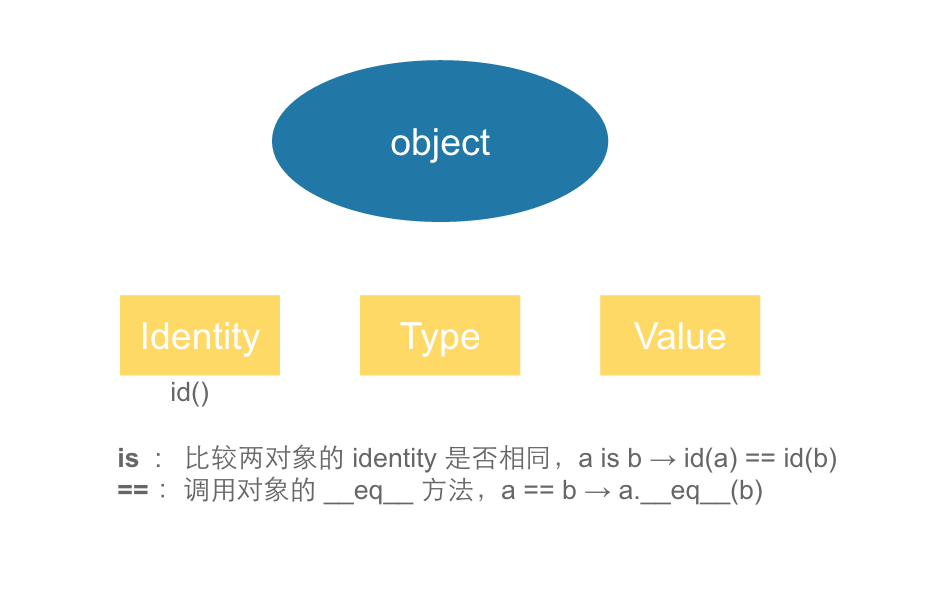# Case 1

``````>>> a = [1, 2]
>>> b = [1, 2]
>>> a == b
True
>>> a is b
False
``````

Everything in Python is an object.
Every object has an identity, a type and a value.

identity 表示对象所在的地址空间，可用 id() 查看：

``````>>> id(a)
4539217360
>>> id(b)
4539217488
``````

# Case 2

``````>>> class A(object):
...     def __eq__(self, other):
...         return True
...
>>> a = A()
>>> a == 1
True
>>> a == 2
True
>>> a == "abcdefg"
True
``````

``````>>> class B(A):
...     def __ne__(self, other):
...         return True
...
>>> b = B()
>>> b == 1
True
>>> b != 1
True
``````

``````+--------+-------------+
| x == y | x.__eq__(y) |
| x != y | x.__ne__(y) |
| x <= y | x.__le__(y) |
| x < y  | x.__lt__(y) |
| x > y  | x.__gt__(y) |
| x >= y | x.__ge__(y) |
+--------+-------------+
``````

# Case 3

``````>>> a = 1
>>> b = 1
>>> a == b
True
>>> a is b
True
>>>
>>> a = 100000
>>> b = 100000
>>> a == b
True
>>> a is b
False
``````

``````if a is None:
...

if a is True:
...
``````